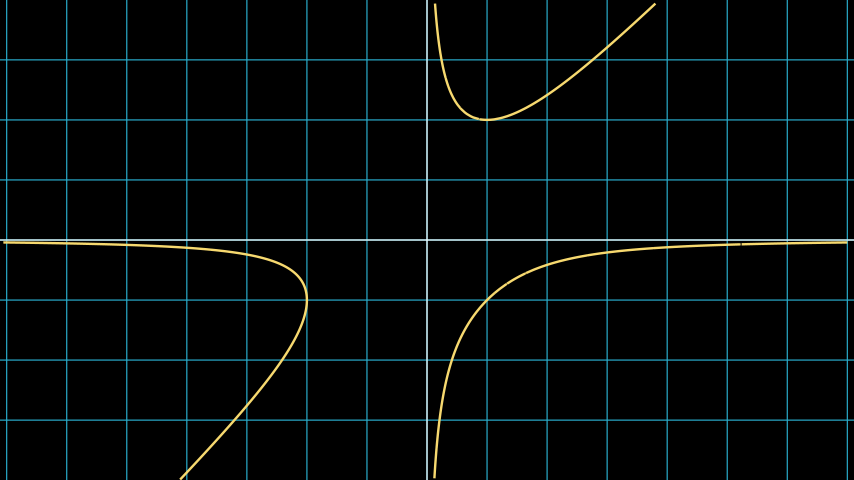# ImplicitFunction#

Qualified name: manim.mobject.graphing.functions.ImplicitFunction

class ImplicitFunction(func, x_range=None, y_range=None, min_depth=5, max_quads=1500, use_smoothing=True, **kwargs)[source]#

Bases: VMobject

An implicit function.

Parameters
• func (Callable[[float, float], float]) – The implicit function in the form f(x, y) = 0.

• x_range (Sequence[float] | None) – The x min and max of the function.

• y_range (Sequence[float] | None) – The y min and max of the function.

• min_depth (int) – The minimum depth of the function to calculate.

• use_smoothing (bool) – Whether or not to smoothen the curves.

• kwargs – Additional parameters to pass into VMobject

Note

A small min_depth $$d$$ means that some small details might be ignored if they don’t cross an edge of one of the $$4^d$$ uniform quads.

The value of max_quads strongly corresponds to the quality of the curve, but a higher number of quads may take longer to render.

Examples

Example: ImplicitFunctionExamplefrom manim import *

class ImplicitFunctionExample(Scene):
def construct(self):
graph = ImplicitFunction(
lambda x, y: x * y ** 2 - x ** 2 * y - 2,
color=YELLOW
)


Methods

 generate_points Initializes points and therefore the shape. init_points Initializes points and therefore the shape.

Attributes

 animate Used to animate the application of any method of self. animation_overrides color depth The depth of the mobject. fill_color If there are multiple colors (for gradient) this returns the first one height The height of the mobject. n_points_per_curve sheen_factor stroke_color width The width of the mobject.
generate_points()[source]#

Initializes points and therefore the shape.

Gets called upon creation. This is an empty method that can be implemented by subclasses.

init_points()#

Initializes points and therefore the shape.

Gets called upon creation. This is an empty method that can be implemented by subclasses.